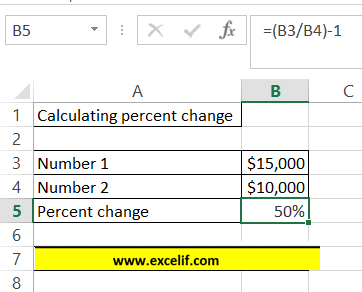## Calculating Percent Change

In this lesson you will learn how to calculate change between two numbers. Thanks to mathematics for sure you know how to calculate percent change. In this article I will show you how to do it in Excel. Calculating percent change with these 2 formulas are really simple in Excel:

• =(new_value/original_value)-1
• =ABS(new_value-original_value)/ABS(original_value)

=(new_value/original_value)-1 is dedicated to beginners. This is really simple formula which you can calculate the percent change of two numbers. Remember that this syntax will give you wrong result when one of number is less than zero.

=ABS(new_value-original_value)/ABS(original_value) is more difficult, but it gives always correct results. I suggest you only use the second formula.

The ABS function returns the absolute value of a number. The absolute value of a number is the number without its sign.

Syntax: =ABS(number)

Number is the real number of which you want the absolute value.

## Example 1 –  using ABS function to calculate percent change

Number1: 8

Number2: 5

Formula is: =(8/5)-1

or

Formula is: =(8-5)/ABS(5)Result is: 60%

Full answer: 8 is 60% greater than 5.

## Example 2 – simple way to calculate percent change

Number1: \$15 000

Number2: \$10 000

Formula is: =(15000/1000)-1

or

Formula is: =(15000-10000)/ABS(10000)Result is: 50%

Full answer: \$15 000 is 50% greater than 10 000.

## Example 3 – more difficult way of calculation percent change

This example is more difficult because one of the number is below zero. Thus you can use only the second formula with ABS function.

Number1: -6 000

Number2: 3 000

Formula is: =(-6000-3000)/ABS(3000)Result is: 300%

Full answer: -6 000 is 300% less than 3 000.

Using such kind of formula will give you always the proper result. No matter if any of numbers will be below zero. I recommend using only this formula to calculate percent change in Excel.

## Tip – percent style formatting

To format Percent Style cell just go to Ribbon > Home > Number and click Percent Style button.## Template

```Further reading: